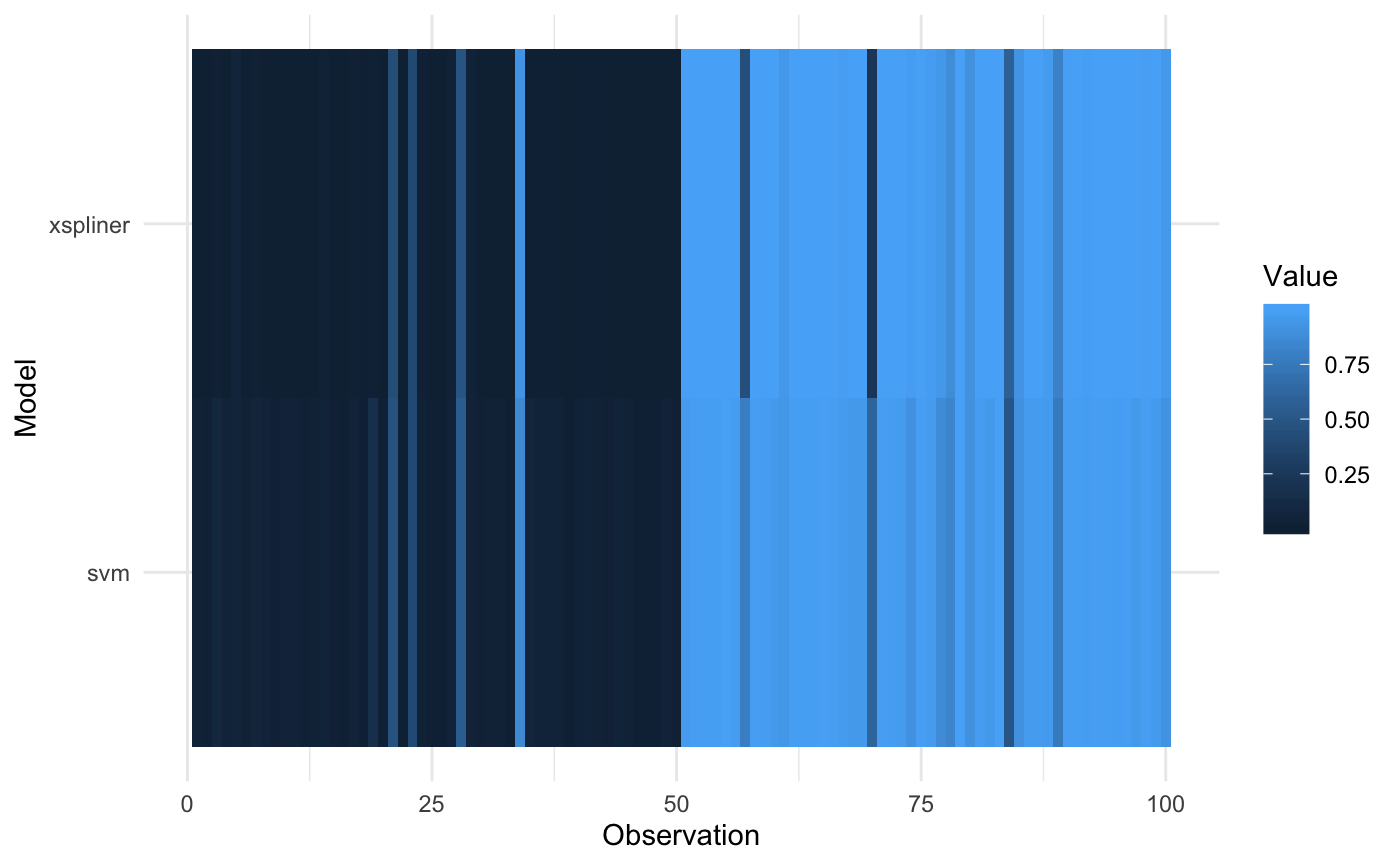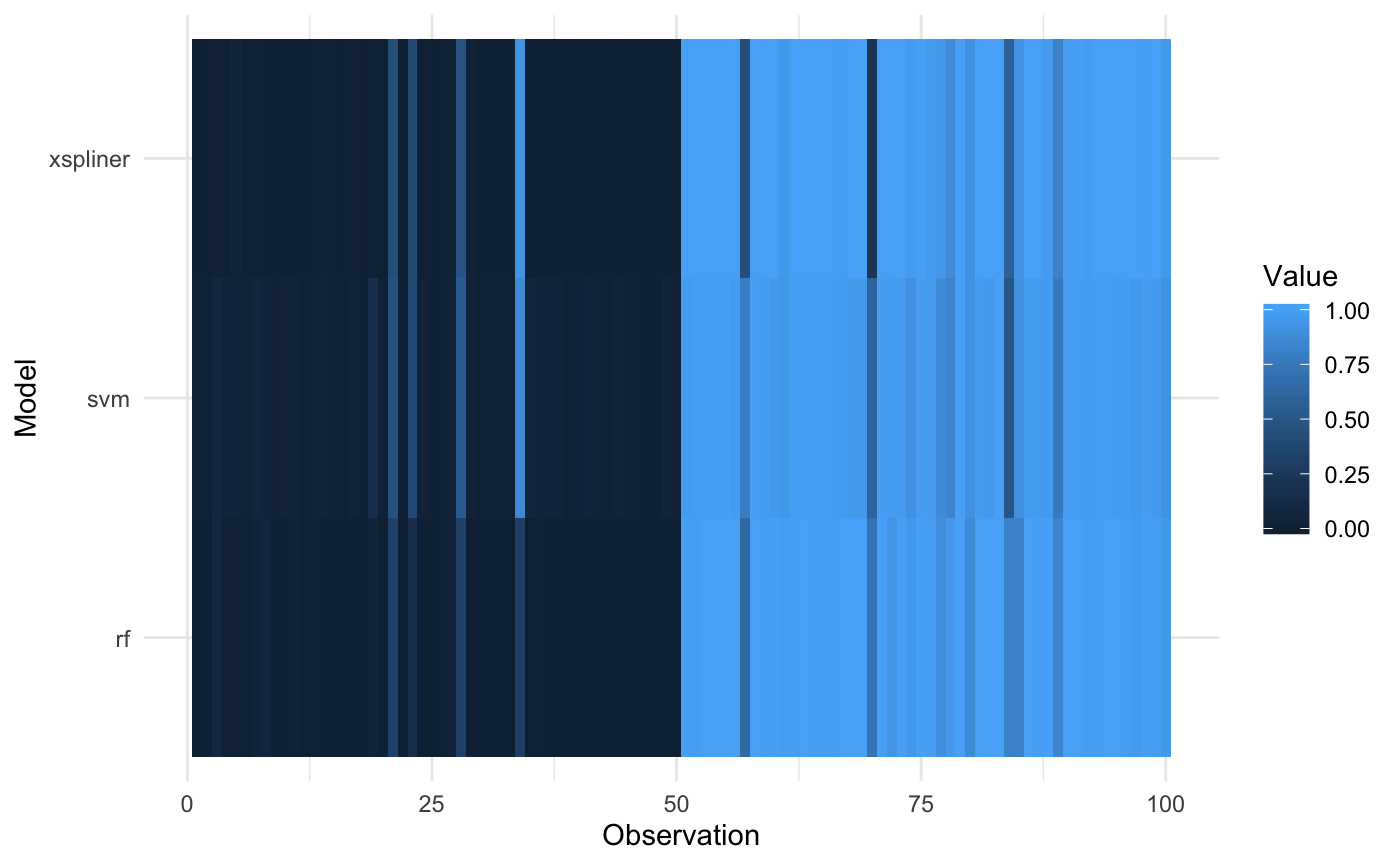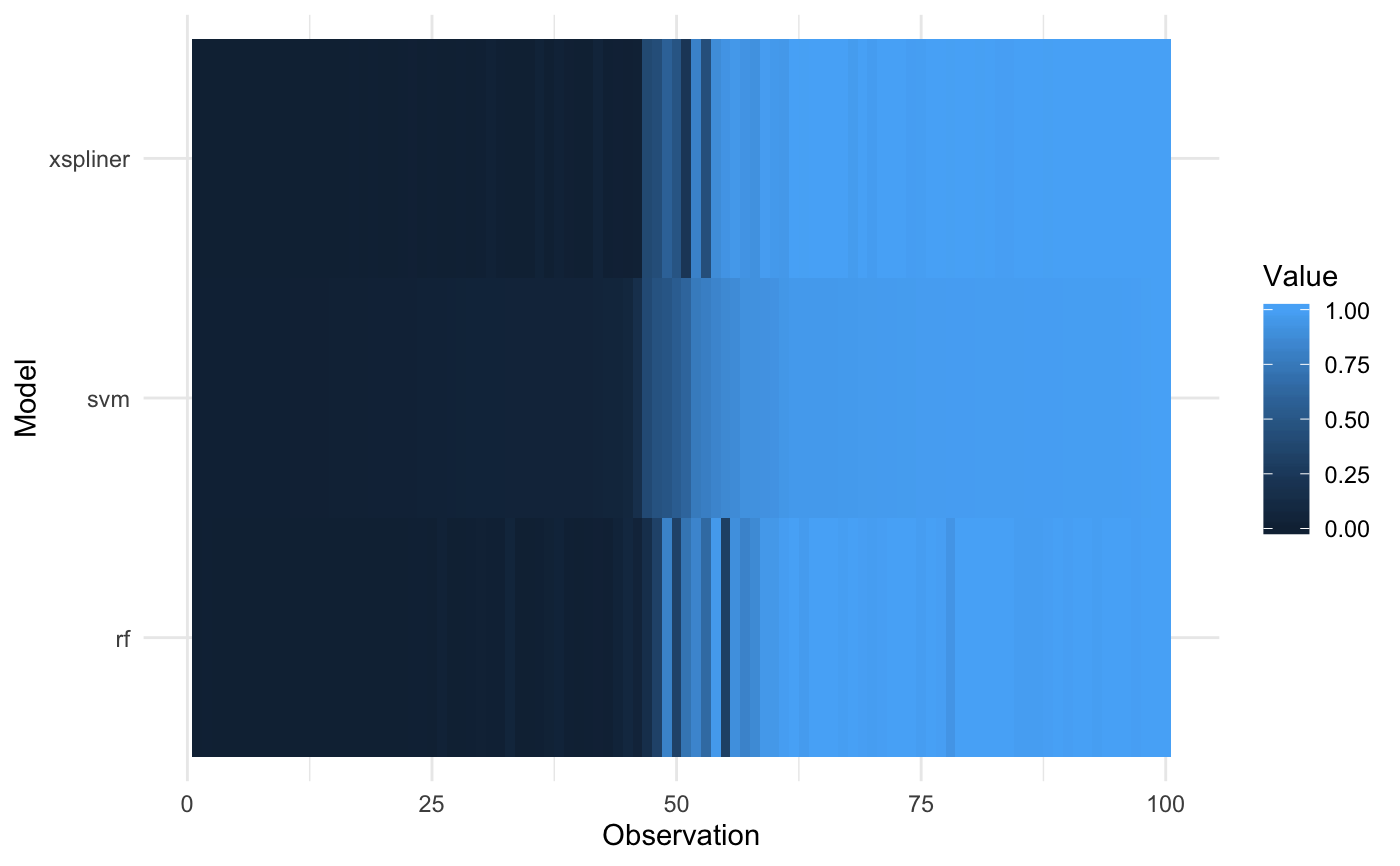The function plots models comparison based on them predictions.

plot_model_comparison(x, model, data, compare_with = list(),
prediction_funs = list(function(object, newdata) predict(object,
newdata)), sort_by = NULL)

## Arguments

x Object of class 'xspliner' Base model that xspliner is based on. Dataset on which predictions should be compared. Named list. Other models that should be compared with xspliner and model. Prediction functions that should be used in model comparison. When comparing models determines according to which model should observations be ordered.

## Examples

iris_data <- droplevels(iris[iris\$Species != "setosa", ])
library(e1071)#> Warning: package ‘e1071’ was built under R version 3.5.2library(randomForest)#> randomForest 4.6-14#> Type rfNews() to see new features/changes/bug fixes.library(xspliner)
# Build SVM model, random forest model and surrogate one constructed on top od SVM
model_svm <- svm(Species ~  Sepal.Length + Sepal.Width + Petal.Length + Petal.Width,
data = iris_data, probability = TRUE)
model_rf <- randomForest(
Species ~  Sepal.Length + Sepal.Width + Petal.Length + Petal.Width,
data = iris_data
)
model_xs <- xspline(
Species ~  xs(Sepal.Length) + xs(Sepal.Width) + xs(Petal.Length) + xs(Petal.Width),
model = model_svm
)
# Prepare prediction functions returning label probability
prob_svm <- function(object, newdata)
attr(predict(object, newdata = newdata, probability = TRUE), "probabilities")[, 2]
prob_rf <- function(object, newdata)
predict(object, newdata = newdata, type = "prob")[, 2]
prob_xs <- function(object, newdata)
predict(object, newdata = newdata, type = "response")

# Plotting predictions for original SVM and surrogate model on training data
plot_model_comparison(
model_xs, model_svm, data = iris_data,
prediction_funs = list(xs = prob_xs, svm = prob_svm)
)# Plotting predictions for original SVM, surrogate model and random forest on training data
plot_model_comparison(
model_xs, model_svm, data = iris_data,
compare_with = list(rf = model_rf),
prediction_funs = list(xs = prob_xs, svm = prob_svm, rf = prob_rf)
)# Sorting values according to SVM predictions
plot_model_comparison(
model_xs, model_svm, data = iris_data,
compare_with = list(rf = model_rf),
prediction_funs = list(xs = prob_xs, svm = prob_svm, rf = prob_rf),
sort_by = "svm"
)